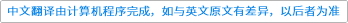International Journal of Mathematics ( IF 0.604 ) Pub Date : 2021-04-10 , DOI: 10.1142/s0129167x2150035x
Xiaojun Wu

In this paper, we obtain a number of results related to the hard Lefschetz theorem for pseudoeffective line bundles, due to Demailly, Peternell and Schneider. Our first result states that the holomorphic sections produced by the theorem are in fact parallel, when the Chern connection associated with the singular metric is computed in the sense of currents, and the corresponding multiplier ideal sheaves are taken into account. Our proof is based on a control of the covariant derivative in the delicate approximation process used in the construction of these sections. Then we show that there is an isomorphism between the space of such parallel sections and the sheaf cohomology group of appropriate degree. As an application, we show that the closedness property of the sections produces a singular holomorphic foliation on the tangent bundle. Finally, we discuss some questions related to the optimality of the multiplier ideal sheaves involved in the generalized hard Lefschetz theorem.down
wechat
bug